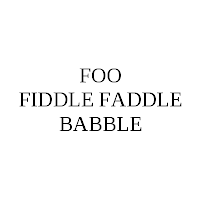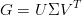Saturday, February 28, 2009

Vectorize Matrix Slice Extraction

There was an interesting thread on the Octave mailing lists recently about vectorizing matrix slice extraction. That is avoiding the dreaded for-loop in something like:

%% create a random sparse matrix
n = 10000; %% matrix dimension
nz = 300; %% number of non-zero elements

ia = floor(rand(nz,1)*n); %% dimension 1 indices
ja = floor(rand(nz,1)*n); %% dimension 2 indices
A(:,:) = sparse(ia, ja, rand(nz,1), n, n, n);

%% taking slices with a for loop, slow, poor scaling to large matrices:
for jj = nz:-1:1
slow_columns(:,jj) = A(:,ja(jj));
endfor

%% example using built-in sub2ind, fast, good scaling to large matrices:
jj = sub2ind(size(A)(1),ja);
fast_columns = A(:,jj);

This is a simplified example, the one in the thread shows how you can access a 5-dimensional matrix with 4 indexes. Knowing the built-in functions that come with your high-level language is well worth it, even if it means you have to do weird things to stay in the high-level language and still get reasonable speed. This allows you to avoid slow looping and use the quick compiled functions that someone else has already spent time and effort developing.

Sunday, February 8, 2009

Unblurring Images

Tikhonov regularization is a pretty cool concept when applied to image processing. It lets you 'de-blur' an image, which is an ill-posed problem. The results won't be as spectacular as CSI, but still cool nonetheless.

OriginalThe original image, CSI fans pretend it is a license plate or a criminal's face in a low quality surveillance video, was made by taking a screenshot of some words in a word processor. The image doesn't have to be character based, but characters make good practice problems.

Blurring

We then blur the image with a Toeplitz matrix. In Octave that's pretty easy:

[m, n] = size(im);

sigma_true = 10;
p = exp(-(0:n-1).^2 / (2 * sigma_true^2))';
p = p + [0; p(n:-1:2)];
p = p / sum(p);

G_true = toeplitz(p);

im = G_true*double(im)*G_true';Generally after doing some floating point operations on an image you won't end up with something still scaled between 0 and 255, so the little function shown below rescales a vector's values to the proper range for a grayscale image.

function im = image_rescale(double_vector)
%% scale values linearly to lie between 0 and 255
m = max(double_vector);
n = min(double_vector);
scale = max(double_vector) - min(double_vector);
im = double_vector - (min(double_vector) + scale/2);
im = im ./ scale;
im = (255 .* im) + 127.5;
endfunction

De-Blurring

This is where the regularization comes in. Since the problem is poorly conditioned we can't just recover the image by straightforward inversion. We'll use the singular value decomposition of our blurring matrix to create a regularized inverse, this is similar to the pseudoinverse. If the singular value decomposition of the blurring matrix isThen the regularized inverse isWhere the entries in the diagonal matrix are given bySo as alpha approaches zero we approach the un-regularized problem. Chapter 4 of Watkins has good coverage of doing SVDs in Matlab. The Octave to perform this process is shown below in the function tik_reg().

function im_tik = tik_reg(im,sigma,alpha)
[m,n] = size(im);
p = exp(-(0:n-1).^2 / (2 * sigma^2))';
p = p + [0; p(n:-1:2)];
p = p / sum(p);

G = toeplitz(p);

[U, S, V] = svd(G,0);

Sinv = S / (S^2 + alpha*eye(length(S),length(S))^2);

im_tik = V*Sinv*U'*double(im)*V*Sinv*U';
endfunction

This gives us two parameters (sigma and alpha) we have to guess in a real image processing problem (because we won't be the ones blurring the original). Another weakness of the method is that we assumed we knew the model that produced the blur, if the real physical process that caused the blur is not similar to Gaussian noise, then we won't be able to extract the original image.

Here's a couple for-loops in Octave that try several combinations of alpha and sigma.

sigma = [9,10,11];
alpha = [1e-6,1e-7,1e-8];

for j = 1:length(sigma)
for i = 1:length(alpha)
unblur = tik_reg(im, sigma(j), alpha(i));
unblur = reshape(image_rescale(reshape(unblur,m*n,1)),m,n);
fname = sprintf("unblur_%d%d.png",i,j);
imwrite(fname, uint8(unblur));
endfor
endforOptical character recognition probably wouldn't work on the output, but a person can probably decipher what the original image said.

Thursday, February 5, 2009

CFD Integrity

Quantifying uncertainty in simulation predictions is something at which most modelling communities fall short, and the Computational Fluid Dynamics community is no exception. There is plenty published on uncertainty estimation, but the state of the practice lags the state of the art considerably in this area.

It does not help matters that it is very hard and expensive to do a thorough job of quantifying the uncertainty of the result of a complex calculation. If it takes a week to get one data point, doing a Monte Carlo sensitivity analysis is probably out of the question, and differentiating your code could take years. It also doesn't help that many of the decision makers who are the consumers of the CFD product don't really want to hear how uncertain the results are, often they want pretty pictures and a warm fuzzy feeling (colorful fluid dynamics is great for marketing too).

I highly recommend the article on quantifying uncertainty by P.J. Roache. It is well written, and it is a special treat to read a CFD paper with a sentence like,

Clearly, we are not interested in such worthless semantics and effete philosophizing, but in practical definitions, applied in the context of engineering and science accuracy.